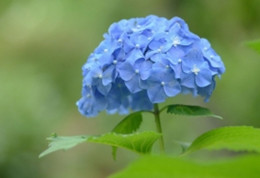# 生辰八字起名，宝宝取名字：孩子起名 姓氏笔画取名组合

4+11画，4+12画，4+13画，4+14画，4+15画，

4+16画，4+17画，5+10画，5+16画，6+10画。

6+15画，9+5画，9+6画，9+7画，9+8画，

9+10画，9+11画，9+12画，9+13画，9+14画，

9+15画，9+16画，9+17画，10+5画，10+6画。

10+11画，10+13画，10+14画，10+15画，

11+10画，11+11画，11+12画，11+13画，

11+14画，11+15画，12+9画，12+10画。

12+11画，12+12画，12+14画，13+8画，

13+10画，13+11画，13+12画，13+13画，

13+14画，13+16画，14+7画，14+9画。

14+10画，14+11画，14+12画，14+15画，

15+14画，17+10画，17+12画，17+16画，

17+17画，17+18画。